Courses

# Shear Force And Bending Moment - 2

## 10 Questions MCQ Test Mock Test Series for Civil Engineering (CE) GATE 2020 | Shear Force And Bending Moment - 2

Description
This mock test of Shear Force And Bending Moment - 2 for Civil Engineering (CE) helps you for every Civil Engineering (CE) entrance exam. This contains 10 Multiple Choice Questions for Civil Engineering (CE) Shear Force And Bending Moment - 2 (mcq) to study with solutions a complete question bank. The solved questions answers in this Shear Force And Bending Moment - 2 quiz give you a good mix of easy questions and tough questions. Civil Engineering (CE) students definitely take this Shear Force And Bending Moment - 2 exercise for a better result in the exam. You can find other Shear Force And Bending Moment - 2 extra questions, long questions & short questions for Civil Engineering (CE) on EduRev as well by searching above.
QUESTION: 1

### Bending moment distribution in a beam is shown in the figure beiow.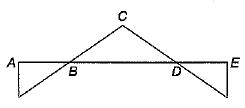The shear force diagram will be given by

Solution:

It is a fixed beam carrying concentrated load at mid span. The shear force diagram will be given by (a).

QUESTION: 2

### A simply supported beam is loaded as shown in the figure. The maximum shear force in the beam will be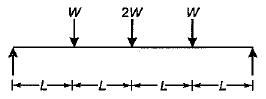Solution: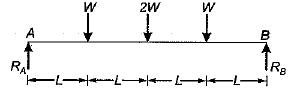The reactions at the supports A and B respectively are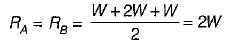The SF diagram will be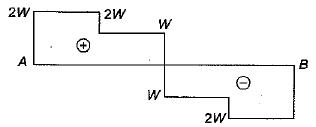The maximum shear force = 2 W.

QUESTION: 3

### The shape of the bending moment diagram for a cantilever beam carrying a uniformly distributed load over its length is

Solution: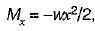which is parabolic in nature.

QUESTION: 4

A simply supported beam is subjected to a distributed loading as shown in the diagram given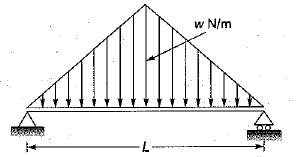What is the maximum shear force in the beam?

Solution: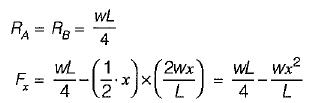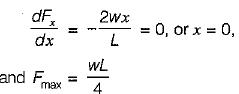QUESTION: 5

The point of contraflexure is a point where

Solution:

At the point of contraflexure, bending moment changes sign.

QUESTION: 6

The ratio of reactions RA and RB of the simply supported beam (as shown) is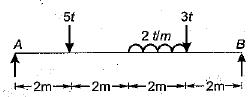Solution: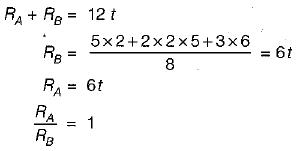QUESTION: 7

The bending moment in a simple supported beam can be calculated with the help of influence line drawn for the following types of load

Solution:
QUESTION: 8

In a simply supported beam of length ‘L’ with a triangular load varying from zero at one end to the maximum value at the other end, the maximum, bending moment is

Solution: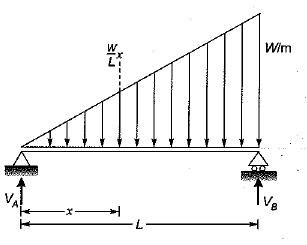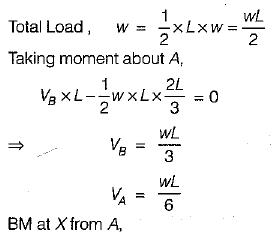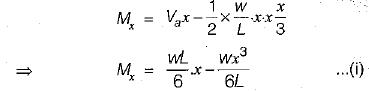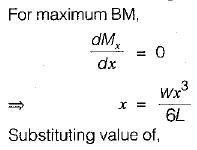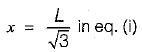we get,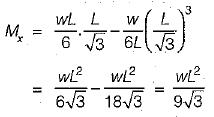QUESTION: 9

For a simply supported beam of length L , the bending moment M is described as M = a (x - x3/L2), 0 ≤ x < L  where a is a constant. The shear force will be zero at

Solution:

Shear force,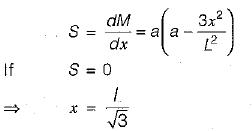QUESTION: 10

Match List-I (Type 0f .be4 rp.with type of loading) with List-ll ( Maximum BM formula) and select the correct answer using the codes given beiowthe lists: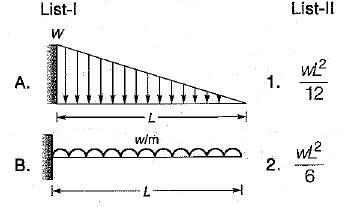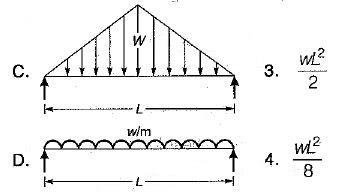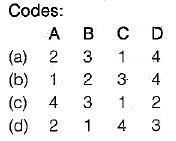Solution: